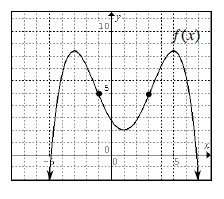### Home > PC > Chapter 7 > Lesson 7.1.1 > Problem7-9

7-9.

Find the regions where each of the following functions is increasing, decreasing, concave up, and concave down. The points of inflection are included on the graph of $f\left(x\right)$ to assist with concavity.

1.Increasing: $\left(–∞, –3\right) ∪ \left(1, 5\right)$
Decreasing: $\left(–3, 1\right) ∪ \left(5, ∞\right)$
Concave Up: $\left(–1, 3\right)$
Concave Down: $\left(–∞, –1\right) ∪ \left(3, ∞\right)$

1. $j ( x ) = \left\{ \begin{array} { c c c } { 2 + 3 x ^ { 2 } } & { \text { if } } & { x \leq 0 } \\ { 2 - x ^ { 2 } } & { \text { if } } & { x > 0 } \end{array} \right.$

Decreasing: $\left(–∞, ∞\right)$
Concave Up: $\left(–∞, 0\right)$
Concave Down: $\left(0, ∞\right)$